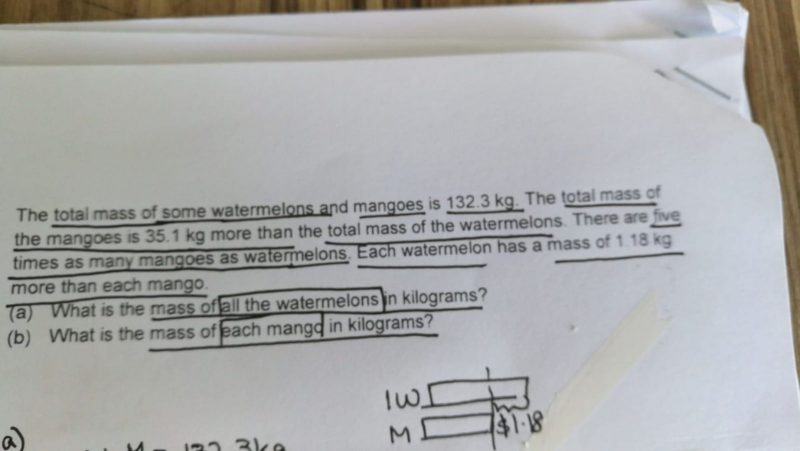# QuestionPlease help with the above mentioned question. Stuck on part (b).

1 Answer

# Answer

Suggested answer:

Total mass = 132.3kg

Total mass for mangoes = (132.3-35.1)kg/2 + 35.1kg = 83.7kg

Total mass for watermelon= (132.3-35.1)kg/2 = 48.6kg

a) Mass of all watermelon is 48.6kg.

Ratio of qty of mangoes to watermelon => 5 : 1

5 unit of mangoes => 83.7kg

1 unit of mangoes => 83.7kg/5 = 16.74kg

1 unit of watermelon =>48.6kg

Diff in mass for 1 unit => 48.6kg – 16.75kg = 31.86kg

No of fruit in 1 unit will be 31.86 / 1.18 = 27.

16.74kg / 27 = 0.62kg

b) Mass of each mango is 0.62kg.

0 Replies 1 Like